Practice the AP 10th Class Maths Bits with Answers Chapter 14 Statistics on a regular basis so that you can attempt exams with utmost confidence.

## AP State Syllabus 10th Class Maths Bits 14th Lesson Statistics with Answers

Question 1.
The mode of the values sin 0°, cos 0°, sin 90° and tan 45° is
A) 0
B) √3
C) 1
D) 
C) 1

Question 2.
Match the following.
Set – A
1. If the median of $$\frac{x}{5}, \frac{x}{3}, \frac{x}{4}$$ is 5, then x =………………
2. Mean of the scores 1 – x, 1, x + 1 is …………………
3. If the mode of x, $$\frac{x}{2}, \frac{x}{2}, \frac{x}{3}, \frac{x}{3}, \frac{x}{3}$$ is 5, then x = …………………

Set – B
(P) 15
(q) 20
(r) 1
A) 1 → r, 2 → p, 3 → q
B) 1 → q, 2 → r, 3 → p
C) 1 → q, 2 → p, 3 → r
D) 1 → p, 2 → r, 3 → q
B) 1 → q, 2 → r, 3 → pQuestion 3.
Median of the scores 1, 3, 5, 7, 9, …………………… 99 is …………………..
A) 51
B) 50
C) 49
D) 48
B) 50

Question 4.
Which one of the following is NOT a measure of central tendency ?
A) Mean
B) Median
C) Range
D) Mode
C) Range

Question 5.
For the terms, x + 1, x + 2, x – 1, x + 3 and x – 2(x ∈ N), if the median of the data is 12, then x = ………………….
A) 9
B) 10
C) 11
D) 13
C) 11

Question 6.
3, 2, 4, 3, 5, 2, x, 6. If the mode of this data is 3, then x = ………………..
A) 4
B) 3
C) 2
D) 5
B) 3

Question 7.
Median of 2, 3, 4, 5, 6, 7 is ……………….
A) 2
B) 5.5
C) 5
D) 4.5
D) 4.5

Question 8.
To elect the leader of your class from 3 contestants, which of the following measures are to be considered ?
A) Mean
B) Mode
C) Median
D) Range
B) Mode

Question 9.
From the given graph of Ogives, median is ………………..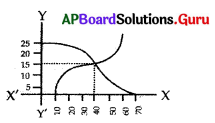A) 15
B) 10
C) 40
D) 20
C) 40Question 10.
Mid values are used to find
A) mean
B) median
C) mode
D) range
A) mean

Question 11.
The mean df first five prime numbers is
A) 4
B) 4.6
C) 5.6
D) 5
C) 5.6

Question 12.
Median of the data 6, 10, 20, x, 12, 14 is 12, then x =
A) 6
B) 10
C) 4
D) 14
B) 10

Question 13.
The A.M of a + 2, a, a – 2 is ……………….
A) a + 2
B) a
C) a – 2
D) 3a
B) a

Question 14.
The midvalue of the class 10 – 19 is ………………….
A) 24.5
B) 20.5
C) 23.5
D) 14.5
D) 14.5

Question 15.
Cumulative frequency are used in ……………… calculations.
A) A.M.
B) median
C) range
D) mode
B) median

Question 16.
While drawing ogive curve we take on X-axis and Y-axis the following respectively.
A) class interval, frequency
B) lower boundary, upper boundary
C) boundaries, cumulative frequency
D) upper boundary, lower boundary
C) boundaries, cumulative frequencyQuestion 17.
The mean of 10 observations is 16.3. By an error, one observation is registered as 32 instead of 23, the correct mean is …………………..
A) 16.1
B) 18.5
C) 15.4
D) 14.8
C) 15.4

Question 18.
The mean of data is. 10. If each observation is multiplied by 2 and 1 is subtracted from each result, the mean of the new observation is ………………..
A) 21
B) 19
C) 9
D) 10
B) 19

Question 19.
The mean of 1, 3, 4, 5, 7, 4 is m. The numbers 3, 2, 2, 4, 3, 3, p have mean m – 1 and median q, then p + q = …………………..
A) 4
B) 3
C) 6
D) 7
D) 7

Question 20.
If the median of the data : 24, 25, 26, x + 2, x + 3, 30, 31, 34 is 27.5, then (Data in the ascending order) is ………………..
A) 27
B) 25
C) 28
D) 30
B) 25

Question 21.
Constructing of a cumulative frequency table is useful in finding………………..
A) median
B) mean
C) mode
D) mean, median, mode
A) median

Question 22.
The abscissa of the point of inter section of less than type and more than type ogive curves gives ………………….
A) median
B) mean
C) mode
D) standard deviation
A) median

Question 23.
The mode of data 3, 3, 4, 5, 3, 5, 6, 3, 7, 9, 11, 7
A) 3
B) 7
C) 3 or 7
D) does not exist
A) 3Question 24.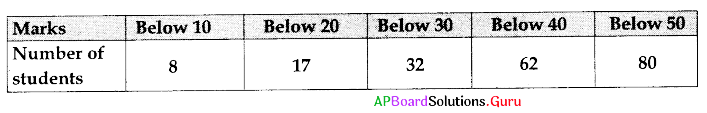In the above distribution the modal class is
A) 10-20
B) 20-30
C) 30-40
D) 40-50
C) 30-40

Question 25.
The mean of n observations X1, X2, ………………., Xn repeated f1, f2, …………….., fn times respectively is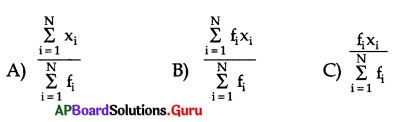D) None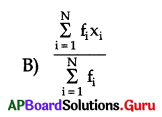Question 26.
The sum of lower limit of median class and upper limit of model class is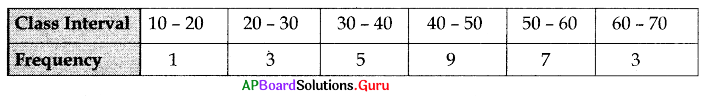A) 60
B) 40
C) 90
D) 50
B) 40

Question 27.
A data has 13 observations arranged in descending order. Which observation presents the median of the data ?
A) 17th
B) 6th
C) 7th
D) None
C) 7th

Question 28.
Cummulative frequency is used to calculate
A) median
B) mode
C) mean
D) None
A) median

Question 29.
Under the usual notations the formula for calculating mode for grouped frequency distribution is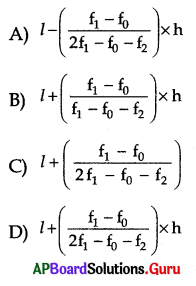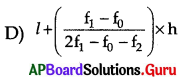Question 30.
Themean of a + 1,a + 3,a + 4 and a + 8 is
A) a + 7
B) a + 4
C) a – 3
D) None
C) a – 3Question 31.
If 5 is added to each and every item of a data, then the arithmetic mean is
A) 5 times to the first arithmetic mean
B) increased by 5 to the first arithmetic mean
C) equal to the first arithmetic mean
D) None
B) increased by 5 to the first arithmetic mean

Question 32.
The median of 24, 20, 32, 18, 28, 16, 25 is
A) 18
B) 16
C) 24
D) 32
C) 24

Question 33.
The median of the following distribution is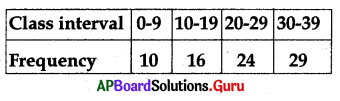A) 23.75
B) 23.25
C) 25.125
D) None
C) 25.125

Question 34.
For the data 9, 8, 7, 7, 6, 3, 7, 2, 1, 7, 9 the mode is
A) 9
B) 7
C) 3
D) 2
B) 7

Question 35.
The modal class of following distribution is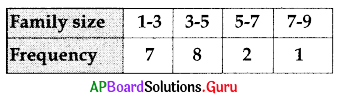A) 1 – 3
B) 3 – 5
C) 5 – 7
D) None
B) 3 – 5

Question 36.
The arithmetic mean of 12, 15, 13, 20, 25 is
A) 17
B) 20
C) 18
D) None
A) 17

Question 37.
The width of the class in the distribution having class intervals 1 – 20, 21 – 40,41 – 60 is
A) 19
B) 19.5
C) 20
D) 20.5
C) 20Question 38.
The arithmetic mean of the following data is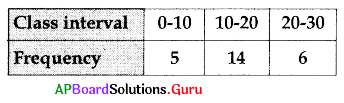A) 18.4
B) 19.4
C) 12.4
D) 15.4
D) 15.4

Question 39.
If mean = 44 and median = 42, then mode =
A) 34
B) 36
C) 38
D) 40
C) 38

Question 40.
Which of the following is more consistent ?
A) AM
B) Median
C) Mode
D) None
A) AM

Question 41.
The mode of 5, $$\frac{x}{2}$$, 6,7, $$\frac{x}{2}$$, 3, $$\frac{x}{2}$$ is 9. Then the value of x is
A) 3
B) 4.5
C) 2
D) 18
B) 4.5

Question 42.
Find the mean of the marks of the students from the given table :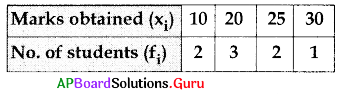A) 20
B) 25
C) 15
D) 10
C) 15

Question 43.
The median of the grouped data can be calculated using the formula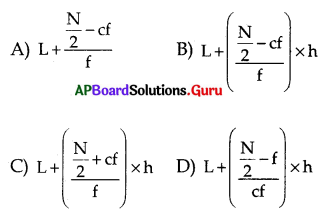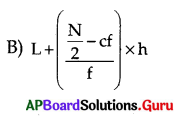Question 44.
The median of 5, 3, 10, 7, 2, 9, 11, 2 is
A) 6
B) 5
C) 7
D) 2
C) 7Question 45.
The median of 5, 6, 9,10, 8,4, 3, 7,11 is
A) 8
B) 7
C) 9
D) 6
D) 6

Question 46.
If the Arithmetic mean of 8, 6,4, x, 3, 6, 0 is 4; then the value of x = ……………..
A) 7
B) 6
C) 1
D) 4
C) 1

Question 47.
Extreme values in the data affect …………………
A) Arithmetic mean
B) Median
C) Mode
D) Range
A) Arithmetic mean

Question 48.
Which of the following is NOT a measure of central tendency ?
A) Range
B) Mode
C) Median
D) Mean
A) Range

Question 49.
Length of the class 11-20 is ………………
A) 9
B) 10
C) 11
D) 20
B) 10

Question 50.
In “More than Ogive curve”, we consider in drawing ……………………
A) more than cumulative frequency, lower limits
B) more than cumulative frequency, upper limits
C) less than cumulative frequency, lower limits
D) less than cumulative frequency, upper limits
A) more than cumulative frequency, lower limits

Question 51.
Observe the following tables :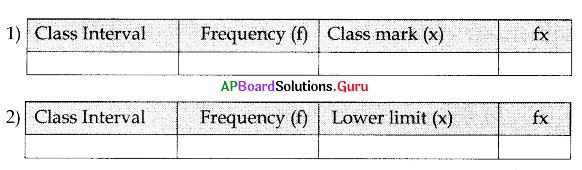For finding Arithmetic Mean by Direct method, the suggested frequency distribution table is ………………..
A) Only (1) is true
B) Only (2) is true
C) (1) and (2) are true
D) None of the above
A) Only (1) is trueQuestion 52.
Median = l + $$\left[\frac{\left(\frac{n}{2}-c f\right)}{f}\right]$$ h, where cf = ………………….
A) Cumulative frequency of the class preceding the median class.
B) Cumulative frequency of the median class.
C) Cumulative frequency of the class succeeding the median class.
D) Sum of the frequencies.
A) Cumulative frequency of the class preceding the median class.

Question 53.
Observe the following graphs.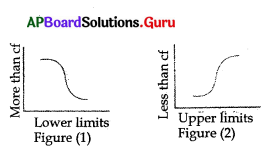Which of the above given figures represents ‘less than’ Ogive curve ?
A) Only (1) is true
B) Only (2) is true
C) Both (1) and (2) are true
D) None of these
B) Only (2) is true

Question 54.
Range of first 5 natural numbersds
A) 0
B) 4
C) 5
D) 9
B) 4

Question 55.
Class interval of 1 – 10 is
A) 8
B) 12
C) 11
D) 10
D) 10

Question 56.
In a data maximum value = x; minimum value = y. Then range
A) x + y
B) x – 1
C) x – y
D) x + 1
C) x – y

Question 57.
Mid value of the class 10 – 20 is
A) 15
B) 13
C) 12
D) 10
A) 15

Question 58.
The class interval of the classes 0 – 7, 7 – 14,14 – 21 ………………….etc.,is
A) 0
B) 7
C) 9
D) 8
B) 7Question 59.
The mean of a – 2, a, a + 2 is …………………
A) 3a
B) $$\frac{\mathrm{a}}{3}$$
C) 1
D) a
D) a

Question 60.
The sum of all deviations taken from Mean =
A) 0
B) -1
C) 1
D) 2
A) 0

Question 61.
A data arranged in descending order has 25 observations. Which observation represents the median ?
A) 15th
B) 14th
C) 13th
D) 12th
C) 13th

Question 62.
The median of 3, 6, 1, 16, 8, 12 is …………………
A) 12
B) 6
C) 9
D) 8
B) 6

Question 63.
Mode of the data 1, 1, 1, 1, 5, 5, 5, 5 is …………………….
A) 5
B) 1
C) 3
D) doesn’t exist
D) doesn’t exist

Question 64.
Mean of 3, 5, 0, x, 11 is 7, then x =
A) 3
B) 4
C) 7
D) 5
C) 7

Question 65.
Range = 25; Maximum value = 30, then the Minimum value is ………………….
A) 9
B) 8
C) 5
D) 7
C) 5Question 66.
The sum of 12 observations is 240, then mean is ……………….
A) 10
B) 12
C 16
D) 20
D) 20

Question 67.
If the mean and mode of a grouped data is 24 and 26.5 respectively then the median is …………………
A) 24. 38
B) 24. 83
C) 23. 48
D) 23. 84
B) 24. 83

Question 68.
The median of the ungrouped data 2, 12, 7, 5, 10 and 6 is
A) 5.5
B) 6
C) 13
D) 6.5
D) 6.5

Question 69.
Median = 52.5; Mean = 54 then mode
A) 49.5
B) 48.5
C) 40. 5
D) 60.1
A) 49.5

Question 70.
Mode = 24.5, Mean = 29.75, then Median = ………………….
A) 28
B) 16
C) 20
D) 82
A) 28

Question 71.
Σ f (x) = 200; n = 20, then $$\overline{\mathbf{x}}$$ =
A) 29
B) 20
C) 16
D) 10
D) 10

Question 72.
The AM of 10 numbers is 20, AM of 30 numbers is 60. AM of combined data is ………………………
A) 40
B) 12
C) 13
D) 50
D) 50

Question 73.
Mean of 8, 6, 4, x, 3, 6, 0 is 4, then x =
A) 0
B) 1
C) 4
D) 14
B) 1Question 74.
Mean of -3, -2, -1,0, 1, 2, 3 is
A) 0
B) -1
C) -2
D) 3
A) 0

Question 75.
The median of 8, 10, 4, 3, 2, 11, 1 is
A) 4
B) 3
C) 7
D) 8
A) 4

Question 76.
The median of scores x1, x2 and 2x1, is 6 and x1 < 2x1 < x2 then x1 = ………………….
A) 7
B) 13
C) 3
D) 6
C) 3

Question 77.
The range of first n natural numbers is …………………
A) n + 1
B) n – 1
C) n
D) 2n -1
B) n – 1

Question 78.
Median of 1 to 9 natural numbers is …………………
A) 5
B) 6
C) 7
D) 4
A) 5

Question 79.
Median of $$\frac{3}{4}, \frac{1}{2}, \frac{2}{3}, \frac{1}{6}, \frac{7}{12}$$ is
A) $$\frac{2}{7}$$
B) $$\frac{7}{9}$$
C) $$\frac{7}{12}$$
D) $$\frac{2}{3}$$
C) $$\frac{7}{12}$$

Question 80.
Mean of a – 2d, a – d, a + d and a + 2d is
A) -a
B) 3a
C) a
D) 5a
C) aQuestion 81.
The mean of first 5 odd multiples of 5 is
A) 15
B) 20
C) 25
D) 35
C) 25

Question 82.
The mean of 10 numbers is 7. The mean of 15 numbers is 12 then the mean of all observations is ……………………
A) 9
B) 10
C) 12
D) 11
B) 10

Question 83.
Mode of 2004, 2005, 2006, ………………… 2015 is ………………….
A) 2004
B) 2015
C) 2009
D) no mode
D) no mode

Question 84.
The sum of the lower limit and upper limit of the modal class from the above distribution table.
A) 90
B) 110
C) 70
D) 50
C) 70

Question 85.
Mean of first ‘n’ natural numbers is ……………………
A) n
B) $$\frac{\mathrm{n}}{2}$$
C) $$\frac{\mathrm{n}-1}{2}$$
D) $$\frac{n+1}{2}$$
D) $$\frac{n+1}{2}$$

Question 86.
The mean of a data is 9. If each observation is multiplied by 3 and then 1 is added to each result, then the mean of new observation is…………………….
A) 19
B) 20
C) 28
D) 30
C) 28

Question 87.
Average of 11 scores is 10.5. If one score 9 is deleted, then the average of the remaining scores is ………………….
A) 10.16
B) 10.06
C) 10.65
D) 13.6
C) 10.65Question 88
……………… is effected by extreme values.
A) Mean
B) Mode
C) Median
D) Mean and Median
A) Mean

Question 89.
If each observation of data is increased by ‘a’ then mean is increases by………………….
A) a
B) $$\frac{a}{2}$$
C) a2
D) a + 1
A) a

Question 90.
To find median, classes should be ……………….
A) of same frequencies
B) of same length
C) continuous
D) discontinuous
C) continuous

Question 91.
For a distribution with odd number (n) of observations, the median is ……………… observation
A) $$\frac{n^{\text {th }}}{2}$$
B) ($$\frac{n+1}{2}$$)th
C) $$\frac{n-1}{2}$$)th
D) $$\frac{\mathrm{n}}{2}$$ – 1) th
B) ($$\frac{n+1}{2}$$)th

Question 92.
Mean of n – observations x1, x2 ……………… xn repeated f1, f2 …………….. fn times is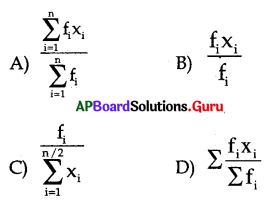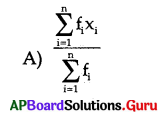Question 93.
In the class 0 – 10,10 – 20,20 – 30,30 – 40 ……………….. lower limit of 10 – 20 is
A) 10
B) 20
C) 30
D) 15
A) 10

Question 94.
Which of the following is not a measure of central tendency ?
A) Mean
B) Range
C) Mode
D) Median
B) RangeQuestion 95.
Which of the following is same as representative of the whole class ?
A) Lower limit
B) Length of the class
C) Upper limit
D) Midpoint
D) Midpoint

Question 96.
The information collected is called
A) Data
B) Mean
C) Median
D) Mode
A) Data

Question 97.
Which of the following measures do you use to find average of marks obtained by a student in the class
A) Mean
B) Median
C) Mode
D) Range
A) Mean

Question 98.
For a symmetrical distribution, which is correct ?
A) Mean > Median > Mode
B) Mean < Median < Mode
C) Mean = Median = Mode
D) $$\frac{\text { Mean }+\text { Median }}{2}$$ = Mode
C) Mean = Median = Mode

Question 99.
For a continuous grouped frequency distribution, the median is given by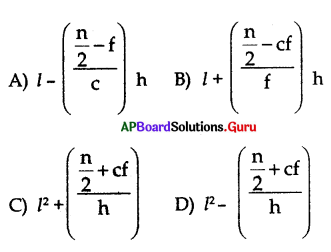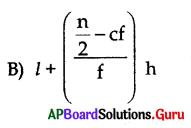Question 100
For a distribution with even number (n) of observations the median is ……………… terms.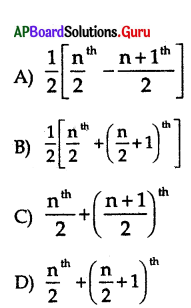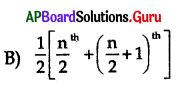Question 101.
Mode of a continuous grouped distribution is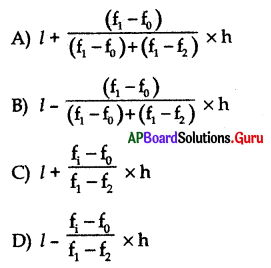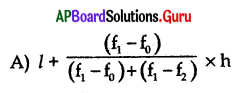Question 102.
If xi and fi are sufficiently small, then which method is an appropriate choice to find mean
A) Assumed – Mean method
B) Step deviation method
C) Direct method
D) Class interval method
C) Direct method

Question 103.
If assumed mean is ‘a’, then mean =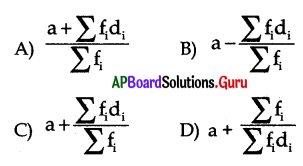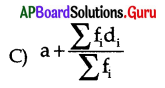Question 104.
In the formula of Mode in the grouped data ‘l’ represents
A) lower limit of the class with highest frequency
B) lower limit of the class with lowest frequency
C) upper boundary of the class with highest frequency
D) lower boundary of the class with low frequency
A) lower limit of the class with highest frequency

Question 105.
………………… of all bars is same in bargraph.
A) Length
B) Width
C) Circle
D) Height
B) Width

Question 106.
In the formula of median in the grouped data ‘l’ represents
A) upper limit of the median class
B) lower limit of the median class
C) upper boundary of the median class
D) lower boundary of the median class
B) lower limit of the median class

Question 107.
The class mark of a class interval is
A) upper boundary + lower boundary
B) upper boundary – lower boundary
C) $$\frac{\text { upper boundary + lower boundary }}{2}$$
D) $$\frac{\text { upper boundary – lower boundary }}{2}$$
C) $$\frac{\text { upper boundary + lower boundary }}{2}$$

Question 108.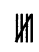|| represents
A) 5
B) 6
C) 7
D) 10
C) 7Question 109
………………… is known as Father of Statistics.
A) Thales
B) Fisher
D) Cayley
D) Thompson
B) Fisher

Question 110.
Number of items in a class interval is called
A) Range
B) Class
C) Frequency
D) Raw data
C) Frequency

Question 111.
Mid values are used to calculate
A) Median
B) Mode
C) Mean
D) Range
C) Mean

Question 112.
Mode is the value of variable which occurs ………….. number of times.
A) Minimum
B) Zero
C) Maximum
D) 2
C) Maximum

Question 113.
We use ……………… to conclude which school has a better performance in an examination.
A) Median
B) Mean
C) Mode
D) Cumulative frequency
B) Mean

Question 114.
Representing data with the help of pictures is called
A) bargraph
B) data
C) pictograph
D) line graph
C) pictograph

Question 115.
Histograms consists of
A) circles
B) rectangles
C) sectors
D) squares
B) rectangles

Question 116.
Pie diagram consists of
A) rectangles
B) squares
C) circles
D) sectors
D) sectorsQuestion 117.
If the mean of x1, x2, x3 ………………… xn is $$\overline{\mathbf{x}}$$ then the mean of $$\frac{\mathbf{x}_{1}}{\mathbf{a}}, \frac{\mathbf{x}_{\mathbf{2}}}{\mathbf{a}}$$ ……………… $$\frac{\mathbf{x}_{\mathrm{n}}}{\mathrm{a}}$$ is
A) a . $$\overline{\mathbf{x}}$$
B) $$\overline{\mathbf{x}}$$ – a
C) $$\frac{\overline{\mathbf{x}}}{\mathbf{a}}$$
D) $$\overline{\mathbf{x}}$$ + a
C) $$\frac{\overline{\mathbf{x}}}{\mathbf{a}}$$

Question 118.
Empirical relation among Mean, Median and Mode is
A) Mode = 3 Median – 2 Mean
B) Mode = 2 Median – 3 Mean
C) Mode = 3 Median – Mean
D) Mode = Median – Mean
A) Mode = 3 Median – 2 Mean

Question 119.
x – coordinate of point of inter-section of the cumulative frequency ogive is
A) Range
B) Median
C) Mean
D) Mode
B) Median

Question 120.
We use ………………. to conclude average IQ (Intelligent Quotient)of a students in a class
A) Median
B) Mean
C) Mode
D) Range
A) Median

Question 121.
Construction of cumulative frequency table is useful in determining the
A) Mean
B) Median
C) Mode
D) Range
B) Median

Question 122.
The mean of the following data is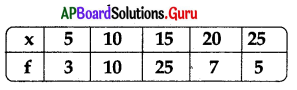A) 15. 0
B) 15. 1
C) 15. 9
D) 16. 2
B) 15. 1

Question 123.
Mode from the following data is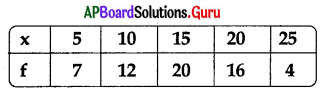A) 5
B) 25
C) 15
D) 10
C) 15Question 124.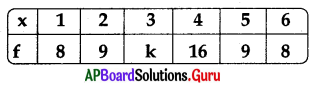The mean of the above data is 3.55 then k =
A) 10
B) 12
C) 31
D) 41
A) 10

Question 125.
The mean class of the following distribution is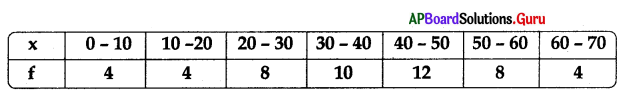A) 60 – 70
B) 50 – 60
C) 40 – 50
D) 30 – 40
D) 30 – 40

Question 126.
For a given data with observation the ‘less than Ogive’ and ‘more than Ogive’ intersect
at (15.5, 20). The median of the data is
A) 20
B) 15.5
C) 10.5
D) 4.5
B) 15.5

Question 127.
The modal class in the following frequency distribution is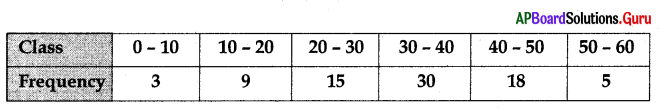A) 30 – 40
B) 40 – 50
C) 50 – 60
D) 20 – 30
A) 30 – 40

Question 128.
The abscissa of the point of inter-section of the less than type and greater than type cumulative frequency curves of a grouped data gives its …………………….
A) Mean
B) Median
C) Mode
D) Range
B) Median

Question 129.
Which of the following graph represents LCF curve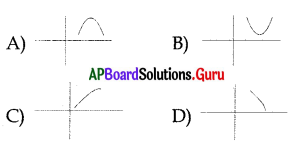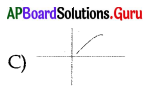Question 130.
Identify GCF curve from the following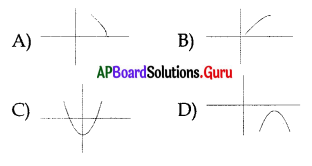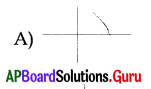Question 131.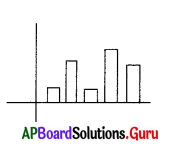A) Histogram
B) Pie chart
C) Picto graph
D) Range
A) HistogramQuestion 132.
In a pie chart we communicate the data with the figures which are in the form of …………………….
A) Circles
B) Sectors
C) Lines
D) Rectangles